Function Repository Resource:

# PolygonMarker

Create markers carefully designed for the creation of publication-quality plots

Contributed by: Alexey Popkov
 ResourceFunction["PolygonMarker"]["name"] returns a unit area Polygon describing the shape "name". ResourceFunction["PolygonMarker"][{p1,…,pn}] returns a unit area Polygon with a shape described by points {p1,…,pn} and a centroid at {0,0}. ResourceFunction["PolygonMarker"][shape, size] returns shape of the specified size. ResourceFunction["PolygonMarker"][shape,{size,angle}] returns shape rotated by angle. ResourceFunction["PolygonMarker"][shape, spec, style] returns a Graphics object suitable for PlotMarkers where the style of shape is defined by style. ResourceFunction["PolygonMarker"][All] returns the list of names of predefined shapes.

## Details and Options

The basic usage syntax is ResourceFunction["PolygonMarker"][shape,spec], where shape is the name of a predefined shape or a list of 2D coordinates describing a simple polygon, and spec can be either size or {size, angle}.
The size can be given as a number or in Scaled or Offset form.
The angle in radians determines the angle of the counterclockwise rotation of shape about its centroid.
ResourceFunction["PolygonMarker"][shape,spec] returns a Polygon graphics primitive that can be used in Graphics.
ResourceFunction["PolygonMarker"][shape,spec,style], where style is a list of graphics directives applied to shape, returns a Graphics object that can be used as a marker for PlotMarkers.
ResourceFunction["PolygonMarker"][shape,spec,style,options] returns a Graphics object with options applied.
The centroid of the Polygon generated by ResourceFunction["PolygonMarker"] is always placed at {0,0} in the internal coordinate system of Graphics.
ResourceFunction["PolygonMarker"][shape,spec,positions], where positions is a list of 2D coordinates and spec contains a numeric specification for size, returns a list of Polygon graphics primitives with centroids placed at positions.
ResourceFunction["PolygonMarker"][shape,spec,positions], where positions is a list of 2D coordinates and spec contains Scaled or Offset specifications for size, evaluates to Translate[ResourceFunction["PolygonMarker"][shape,spec],positions]. It represents a collection of multiple identical copies of shape with centroids placed at positions.
With an Offset size specification, the plot marker has a fixed size specified in printer's points, independent of ImageSize.
ResourceFunction["PolygonMarker"][shape,size] returns a Polygon with area size2 in the internal coordinate system of Graphics. ResourceFunction["PolygonMarker"][shape,Offset[size]] returns a Polygon with area size2 square printer's points. The area taken by the boundary of the returned Polygon is not taken into account.
ResourceFunction["PolygonMarker"][All] and ResourceFunction["PolygonMarker"][] both return the complete list of names of predefined shapes.

## Examples

### Basic Examples (6)

Create a unit area Polygon object of "TripleCross" type rotated counterclockwise by π/6:

 In:=Out=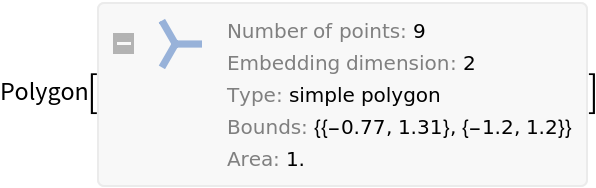The centroid of the polygon is placed at {0,0}:

 In:=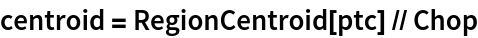Out=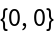Visualize the polygon and its centroid:

 In:=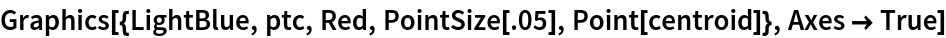Out=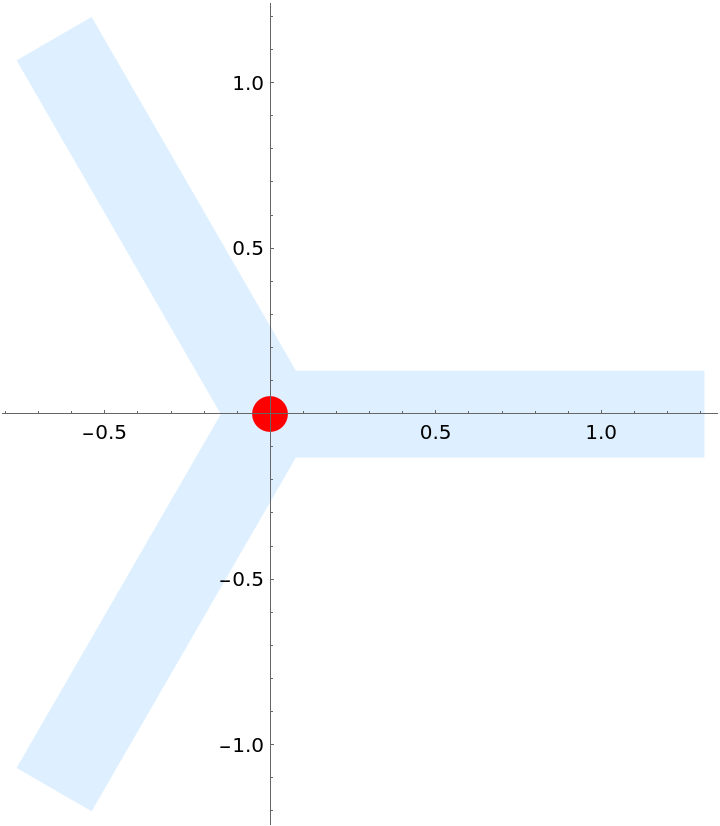Create a displaced Graphics marker in one step:

 In:=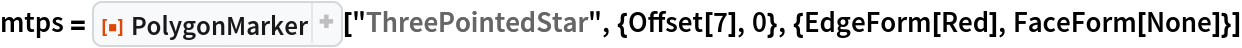Out=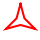This marker is set up to be suitable for PlotMarkers:

 In:=Out=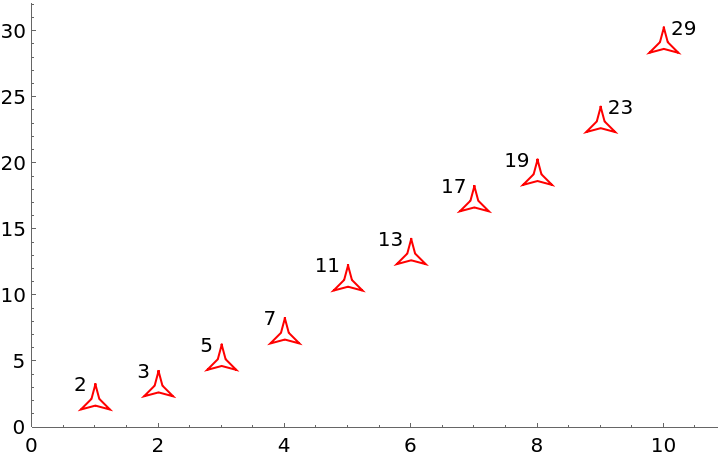Get the complete list of built-in named shapes:

 In:=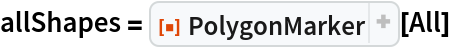Out=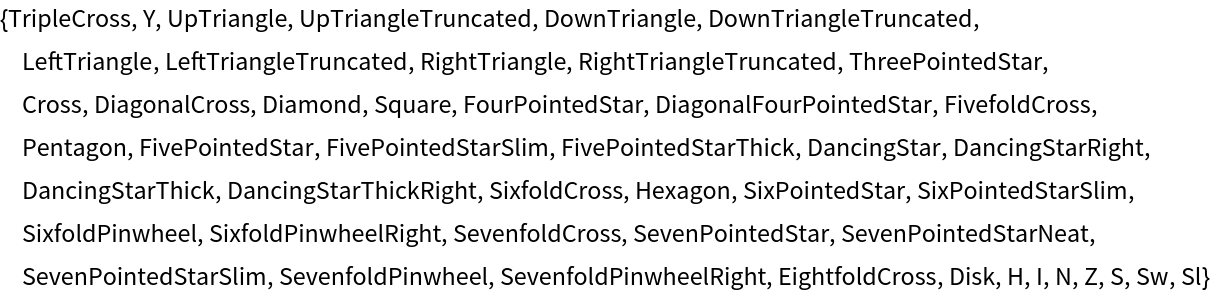Make a Grid of buttons with the shapes for easy selection:

 In:=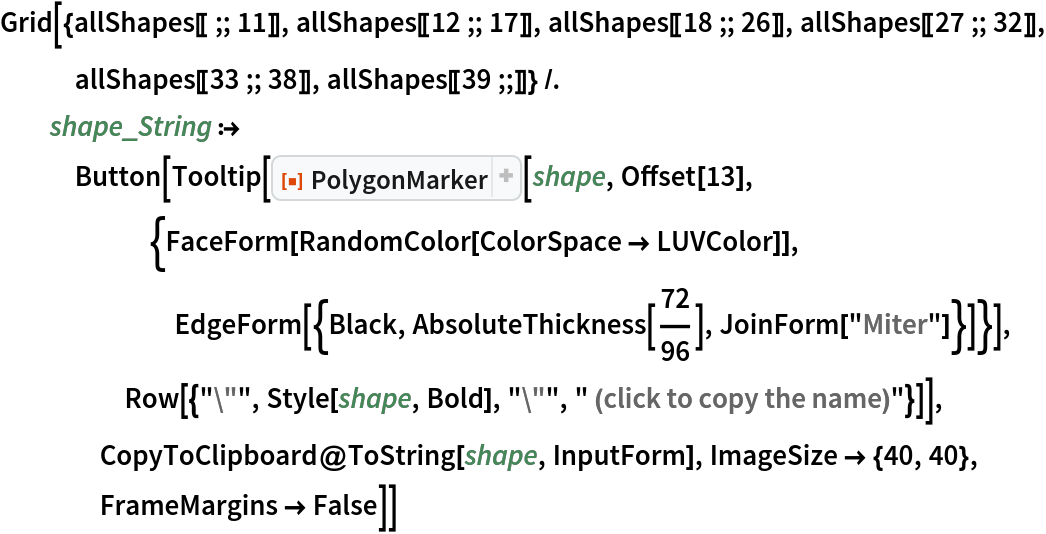Out=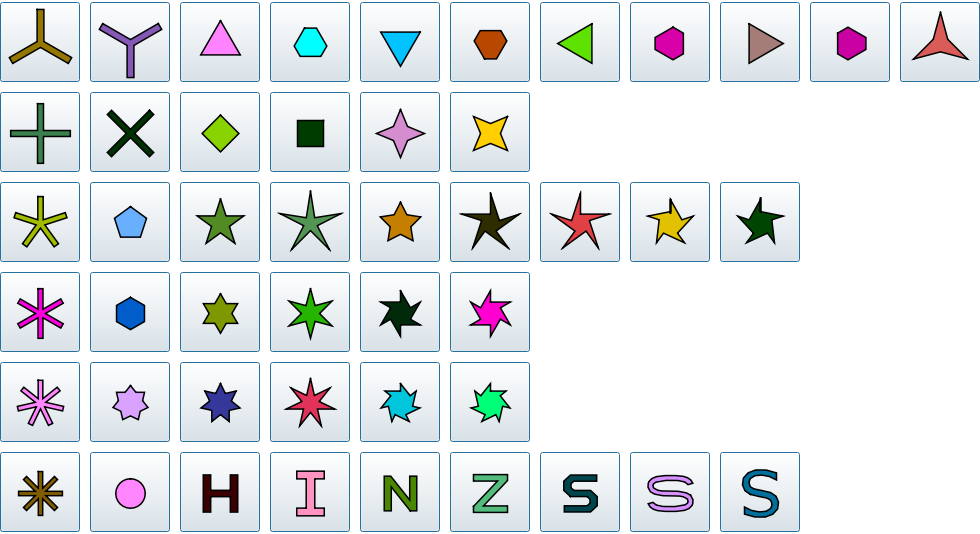Filled markers that pick up PlotStyle and PlotTheme automatically:

 In:=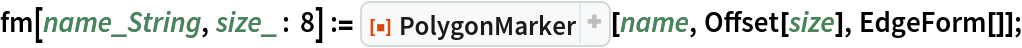In:=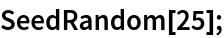In:=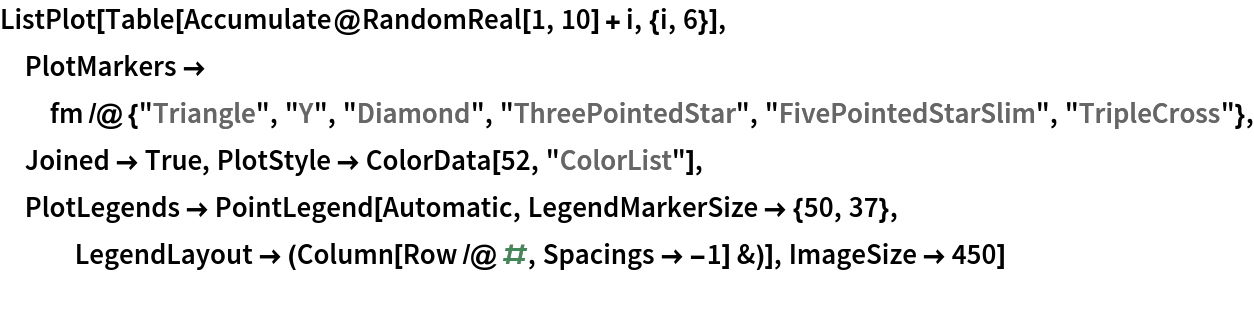Out=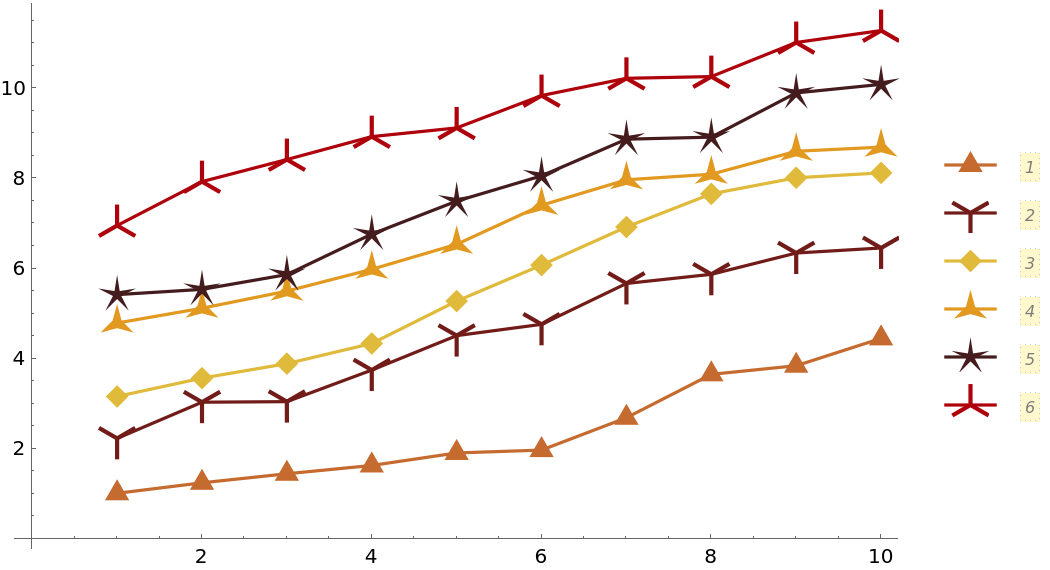In:=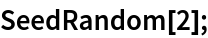In:=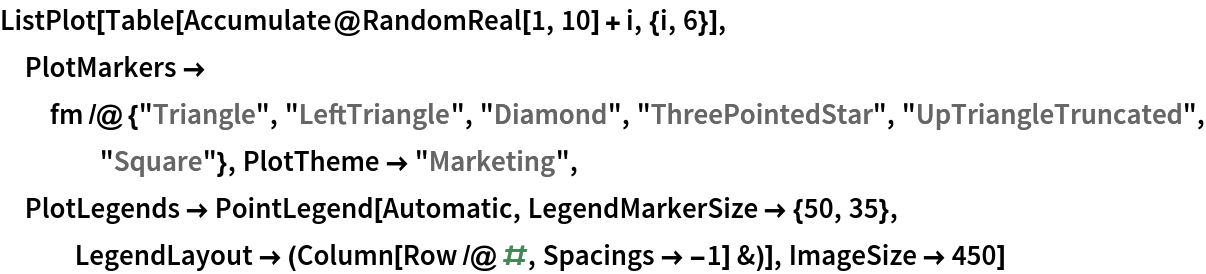Out=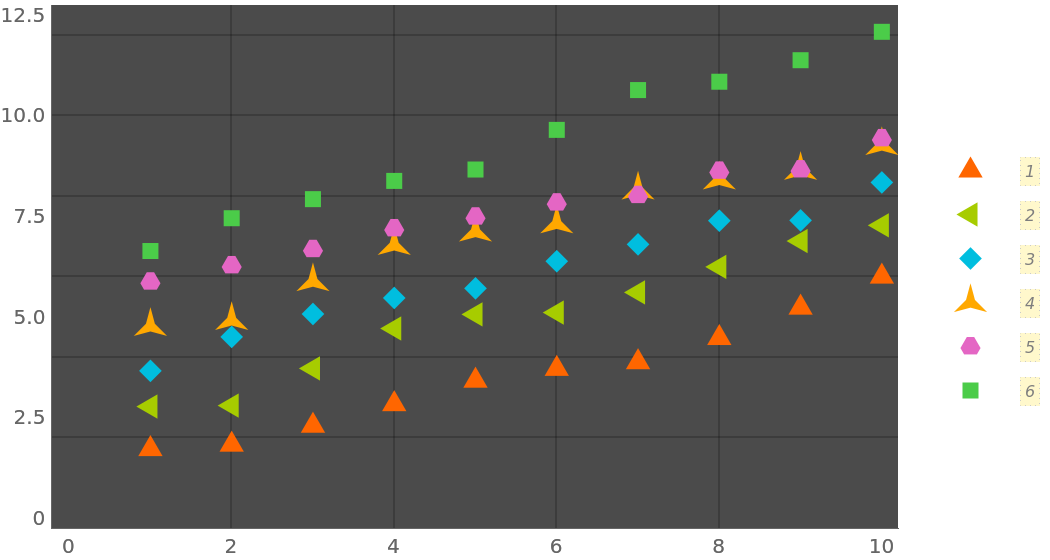Empty markers that pick up PlotStyle and PlotTheme automatically:

 In:=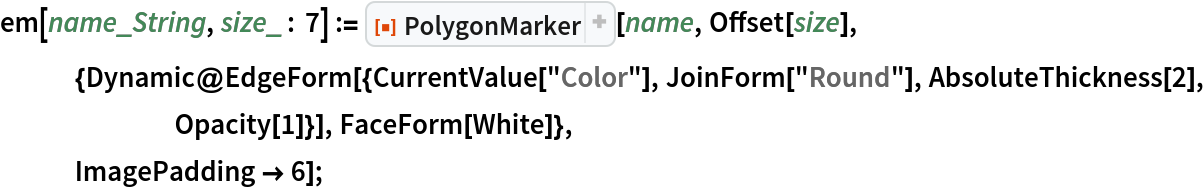In:=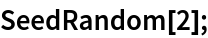In:=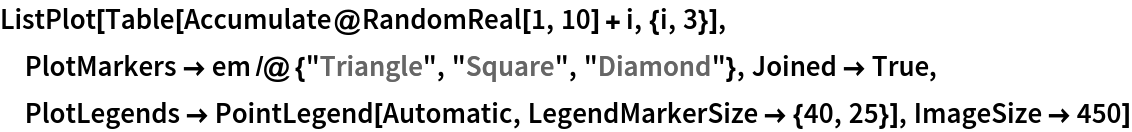Out=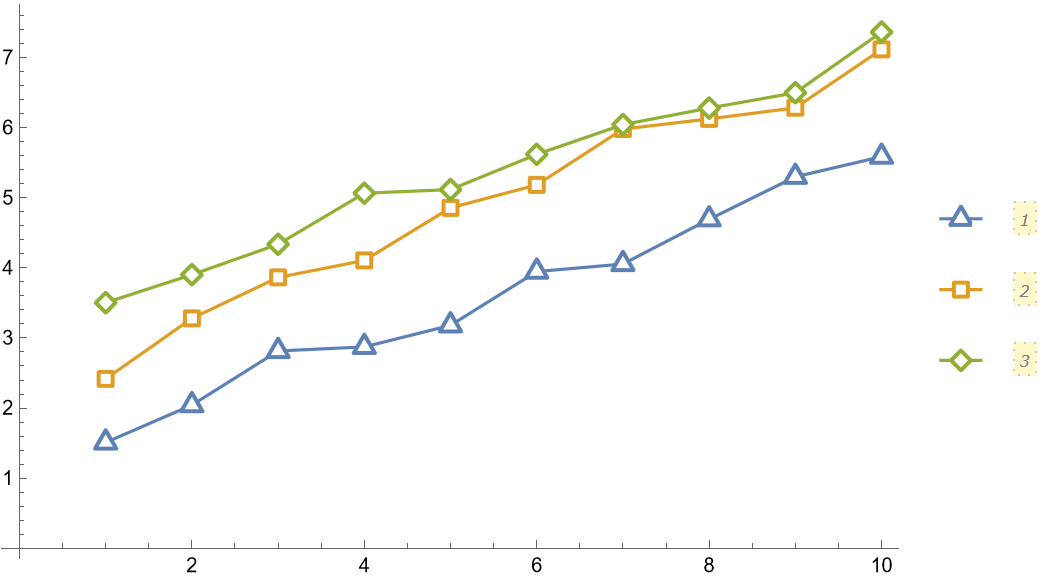In:=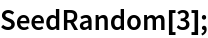In:=Out=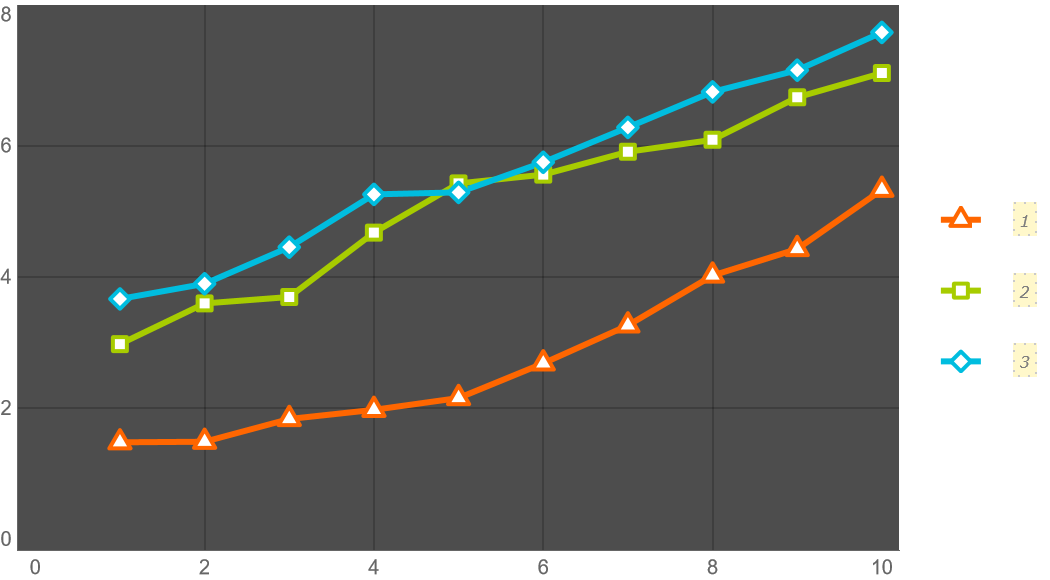Filled markers with lighter filling colors:

 In:=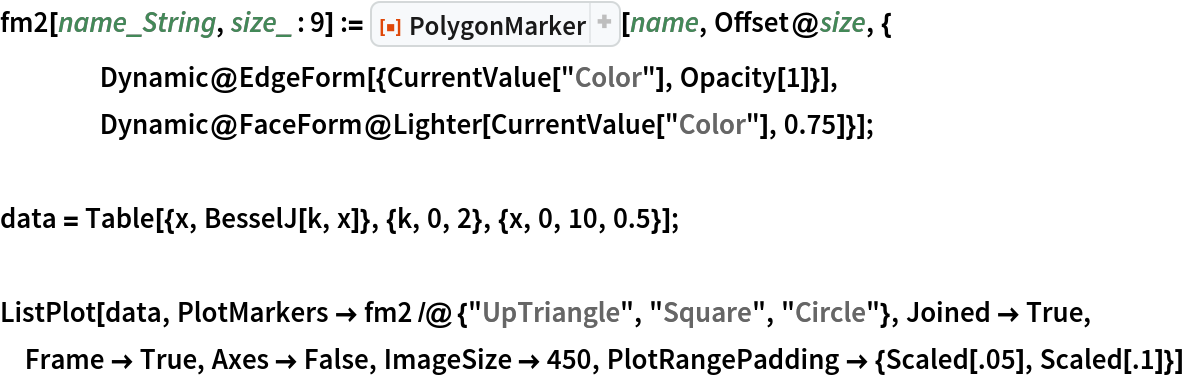Out=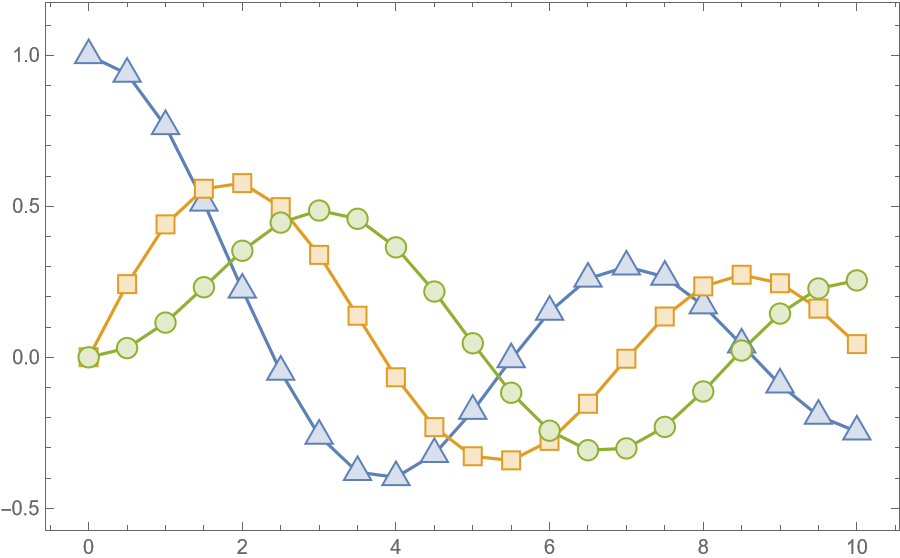### Scope (4)

Use the third argument of PolygonMarker to specify the coordinate(s) where the marker should be placed:

 In:=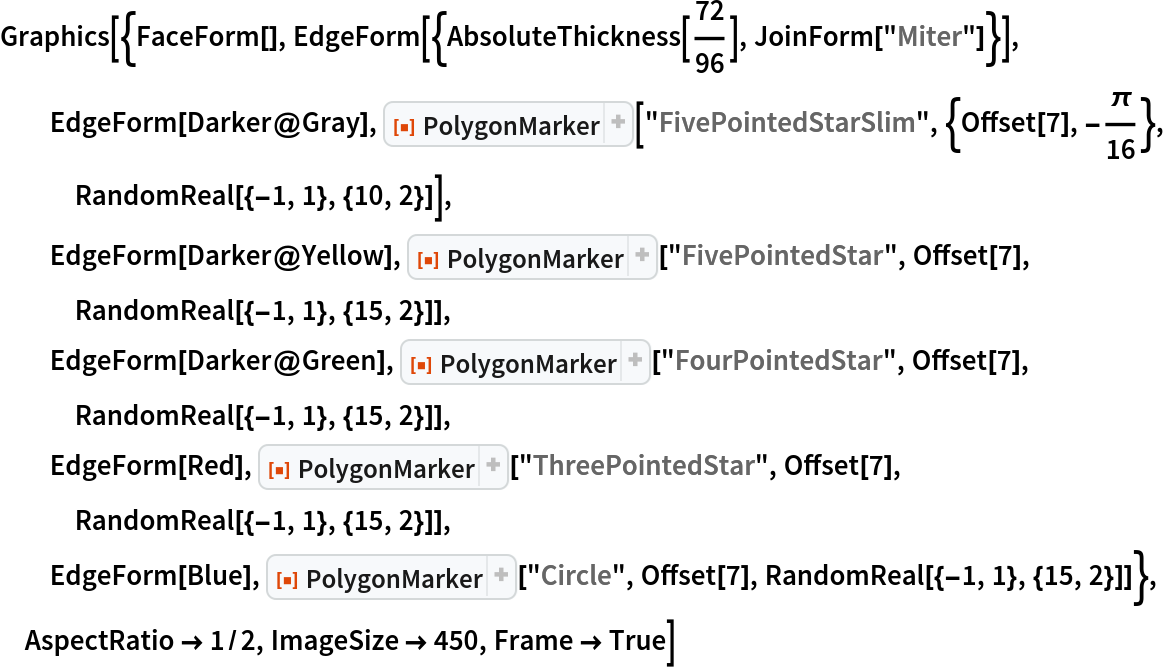Out=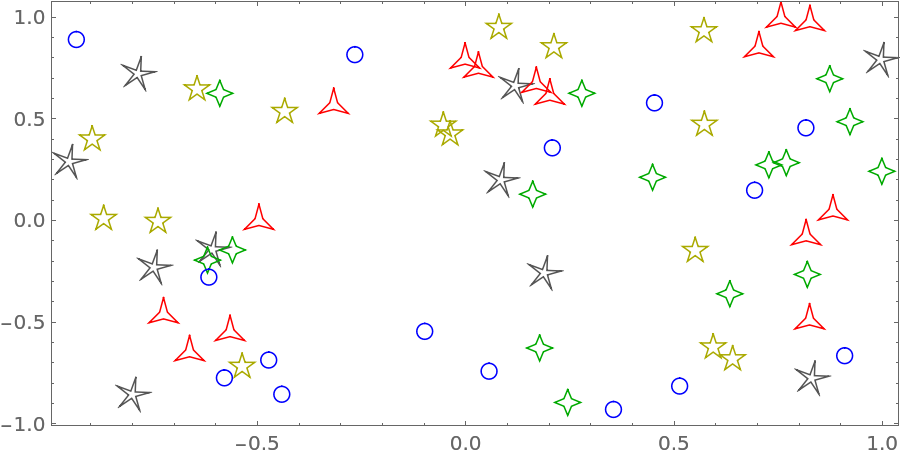Construct a list plot directly from graphics primitives:

 In:=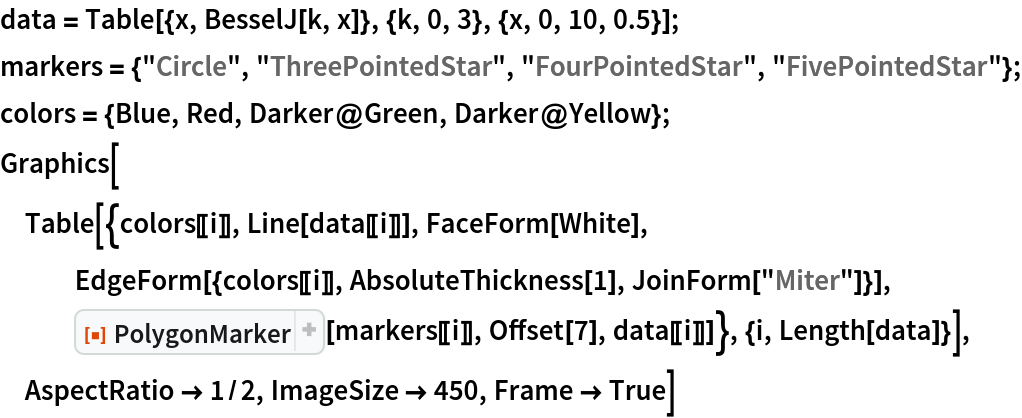Out=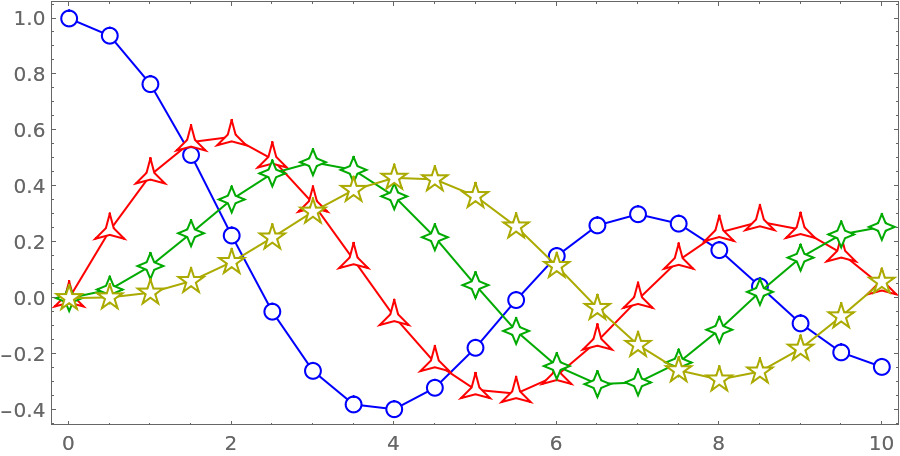Construct a custom list plot where open plot markers have transparent faces for each other (but not for the plotted lines):

 In:=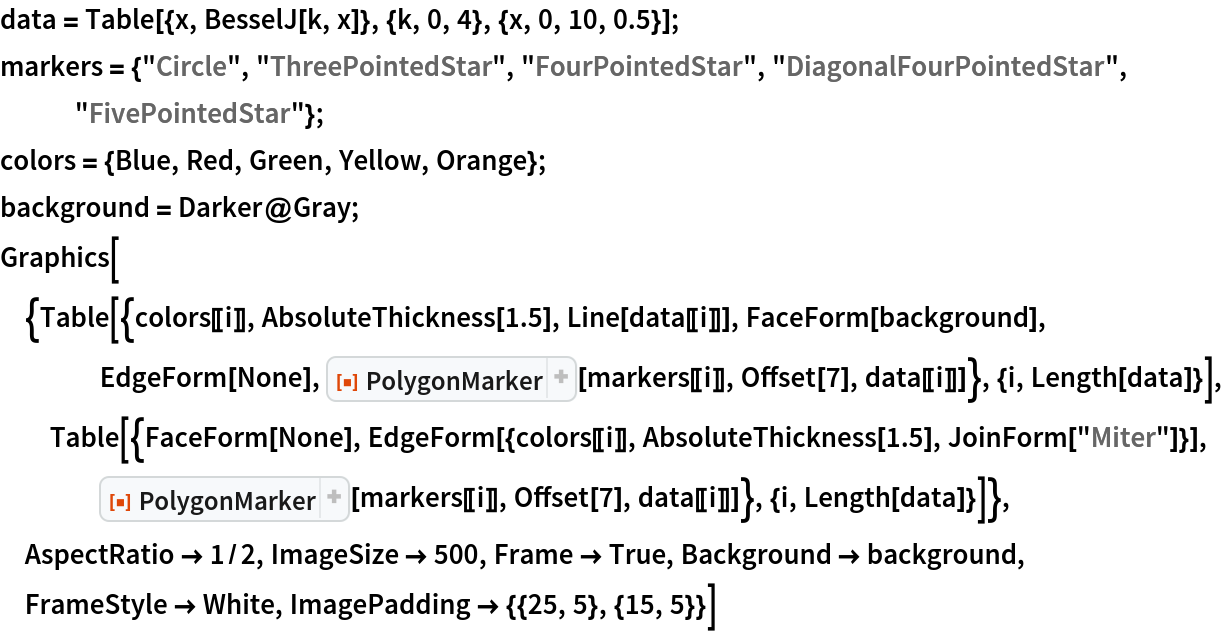Out=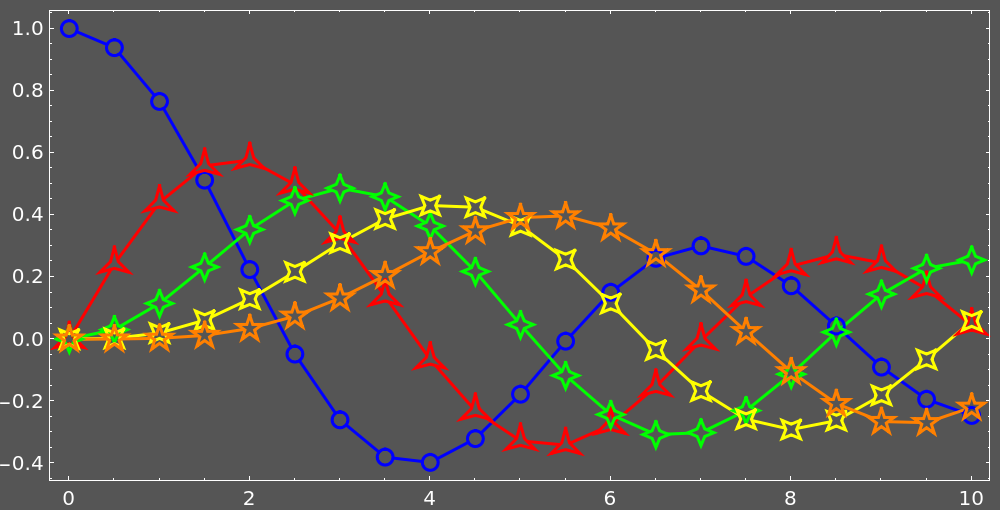Construct a custom list plot where the plot lines do not go through the plot markers (the corresponding portions of the lines are explicitly removed). Other objects like grid lines and background are visible through the markers.

First, define auxiliary functions:

 In:=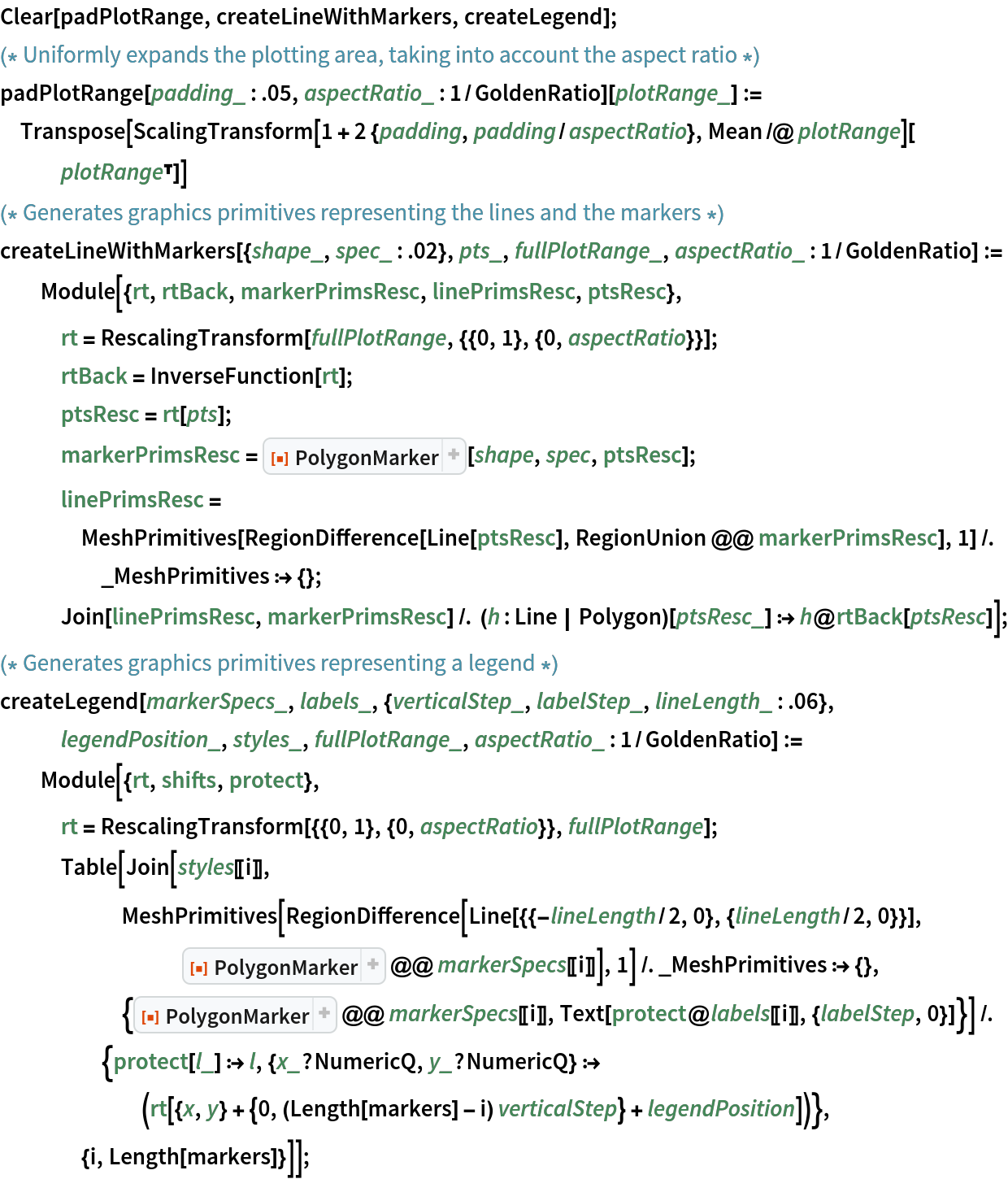Input:

 In:=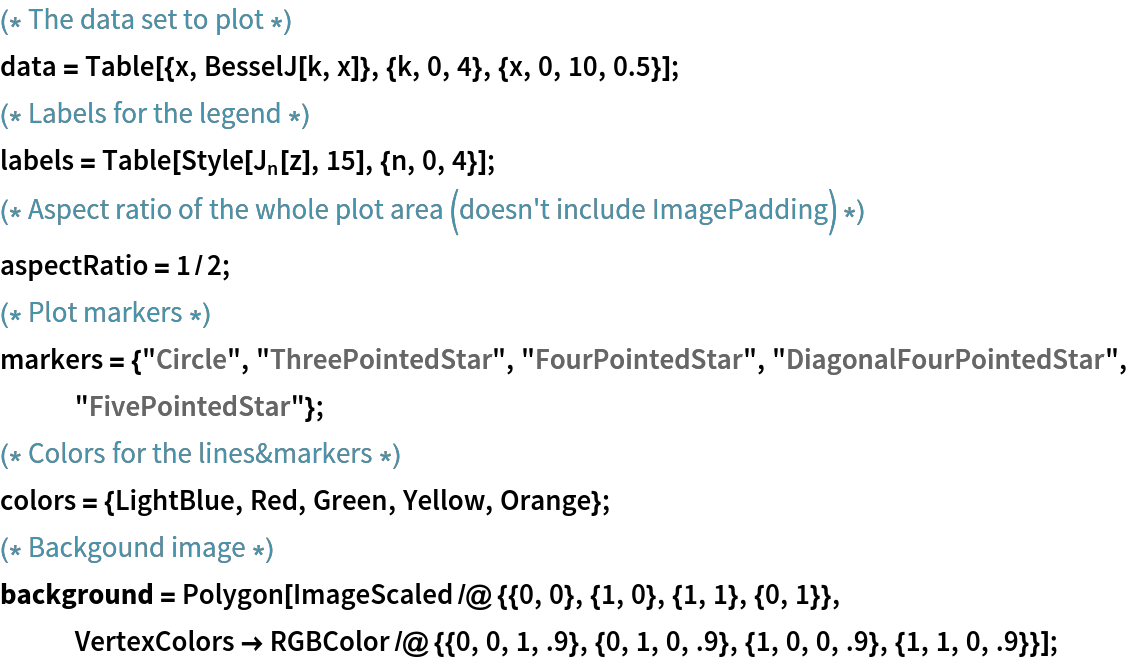Plotting:

 In:=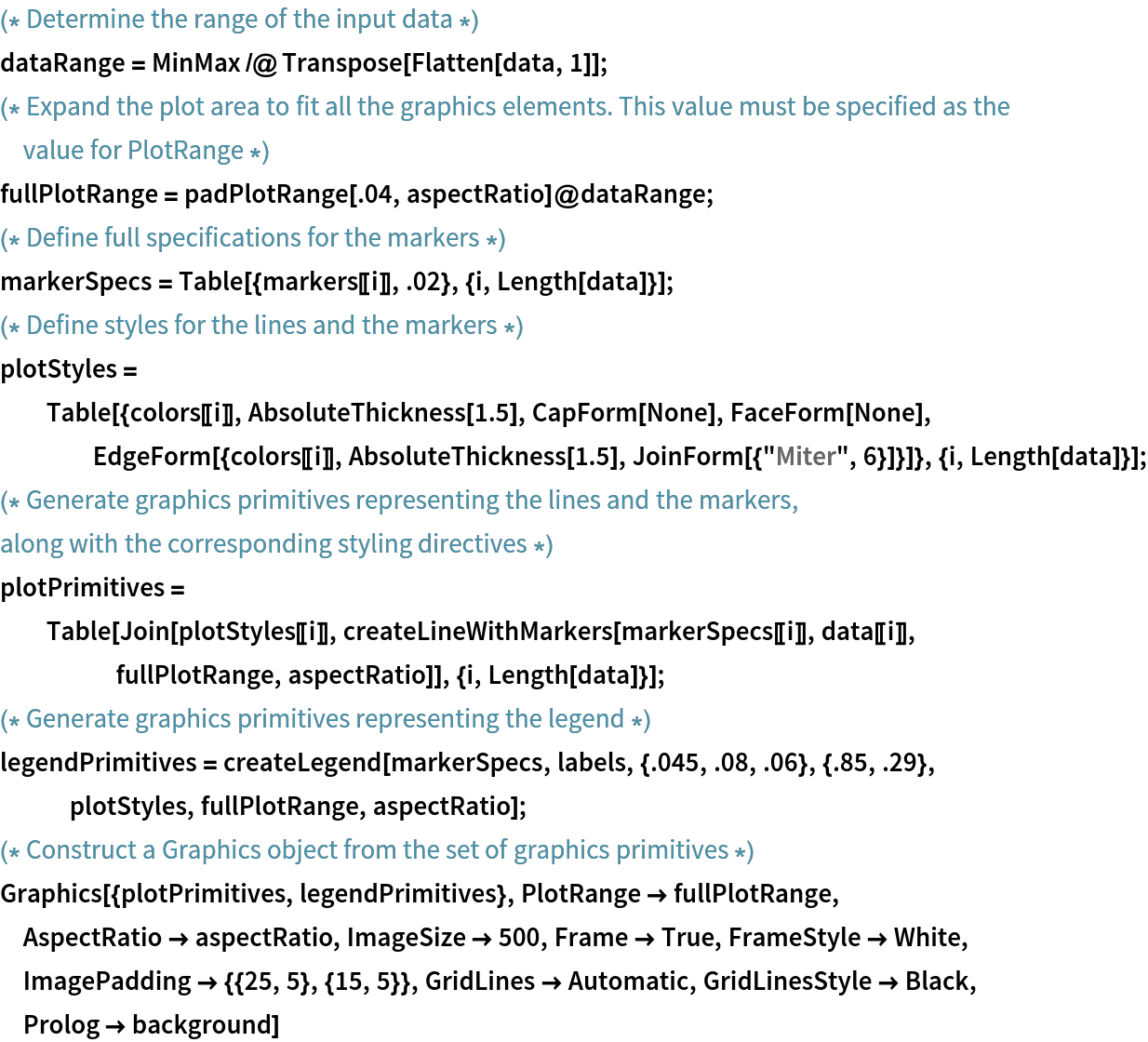Out=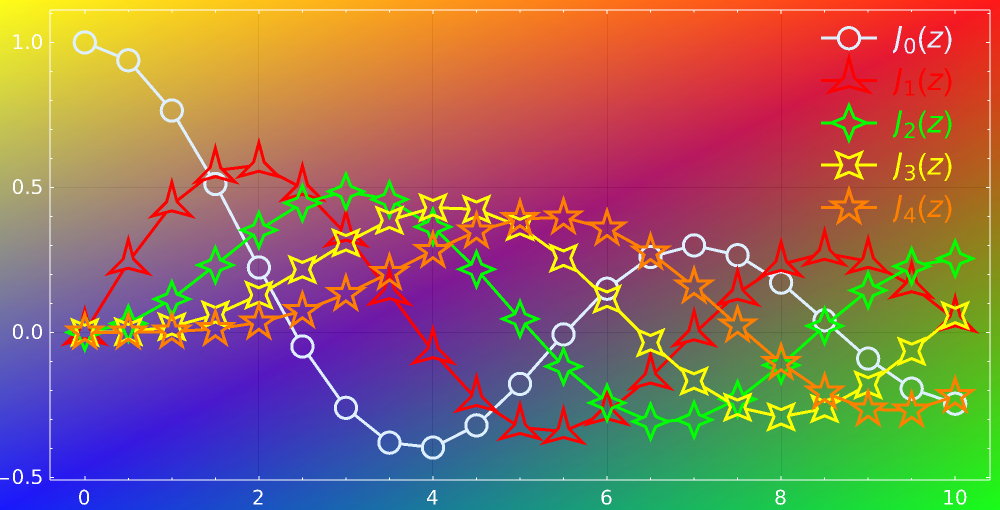### Neat Examples (5)

Center markers that pick up PlotStyle and PlotTheme automatically:

 In:=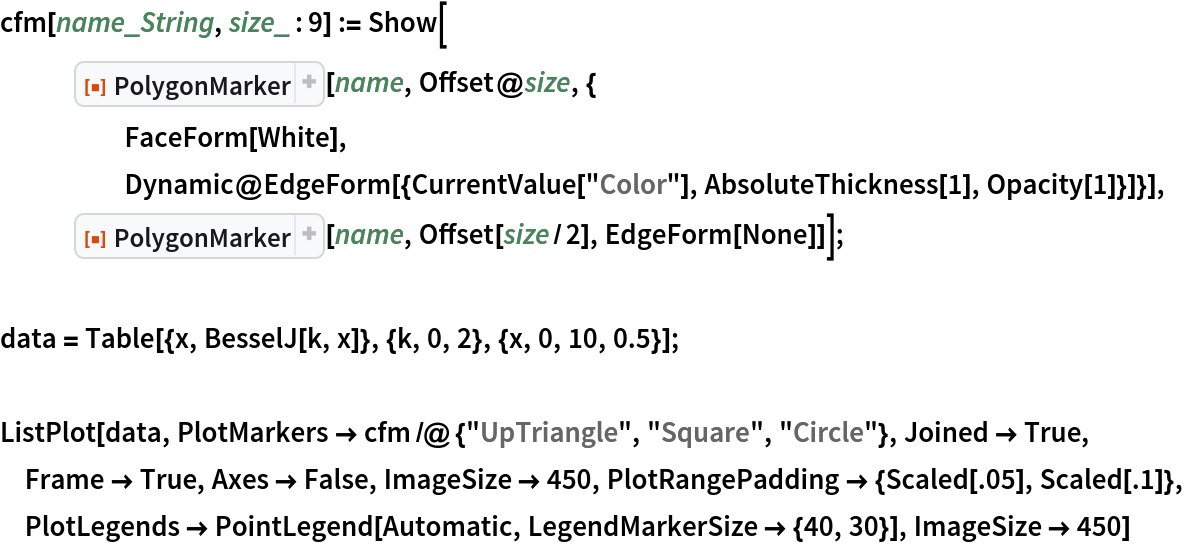Out=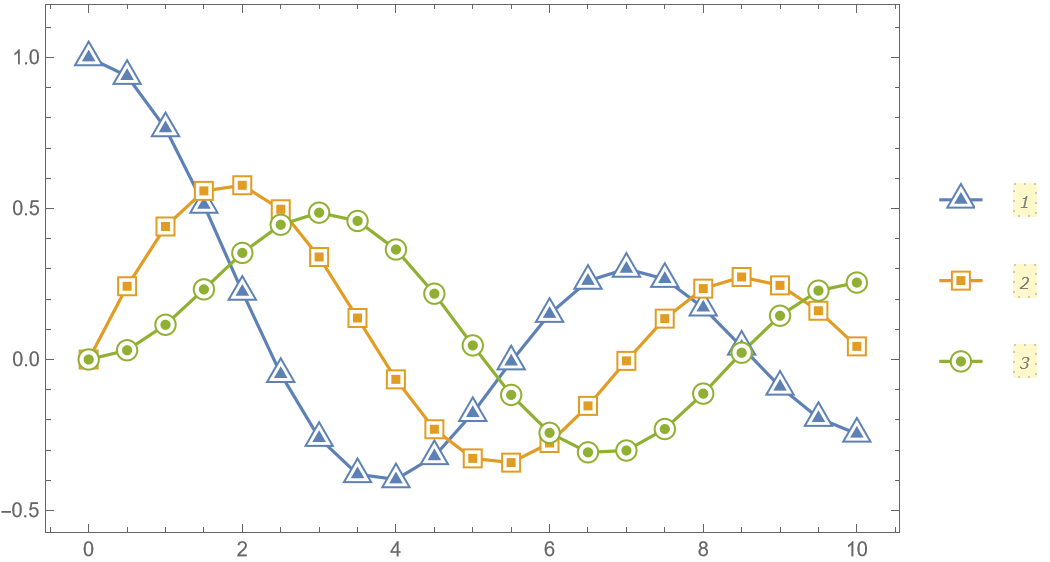Half-filled markers that pick up PlotStyle and PlotTheme automatically:

 In:=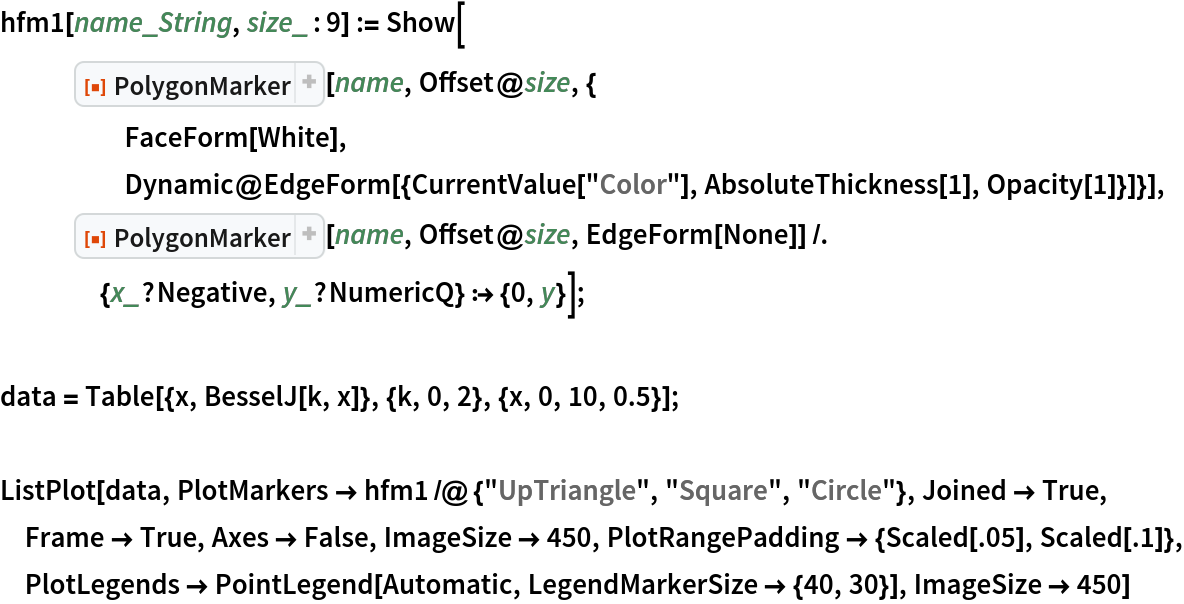Out=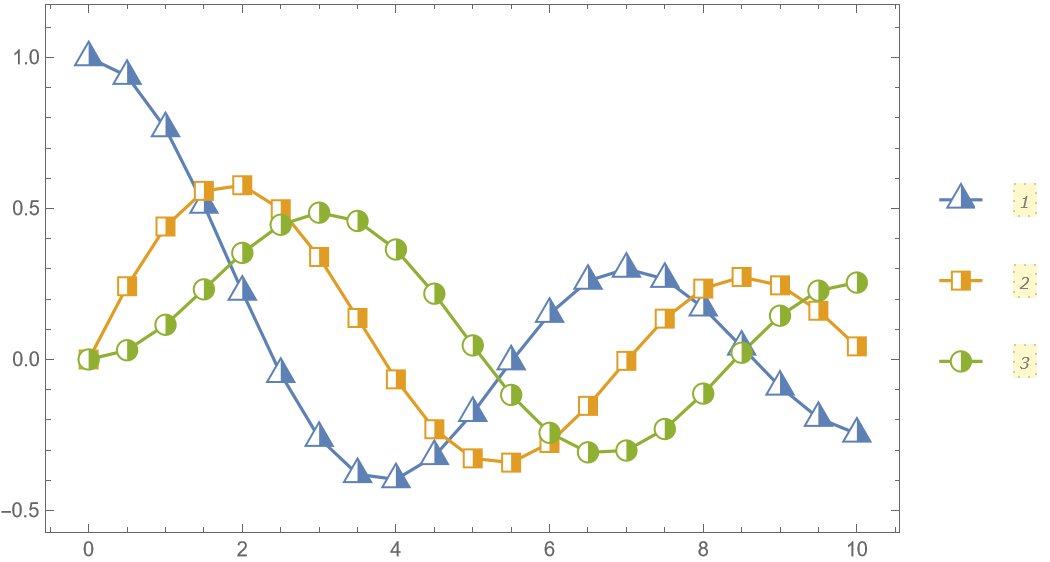In:=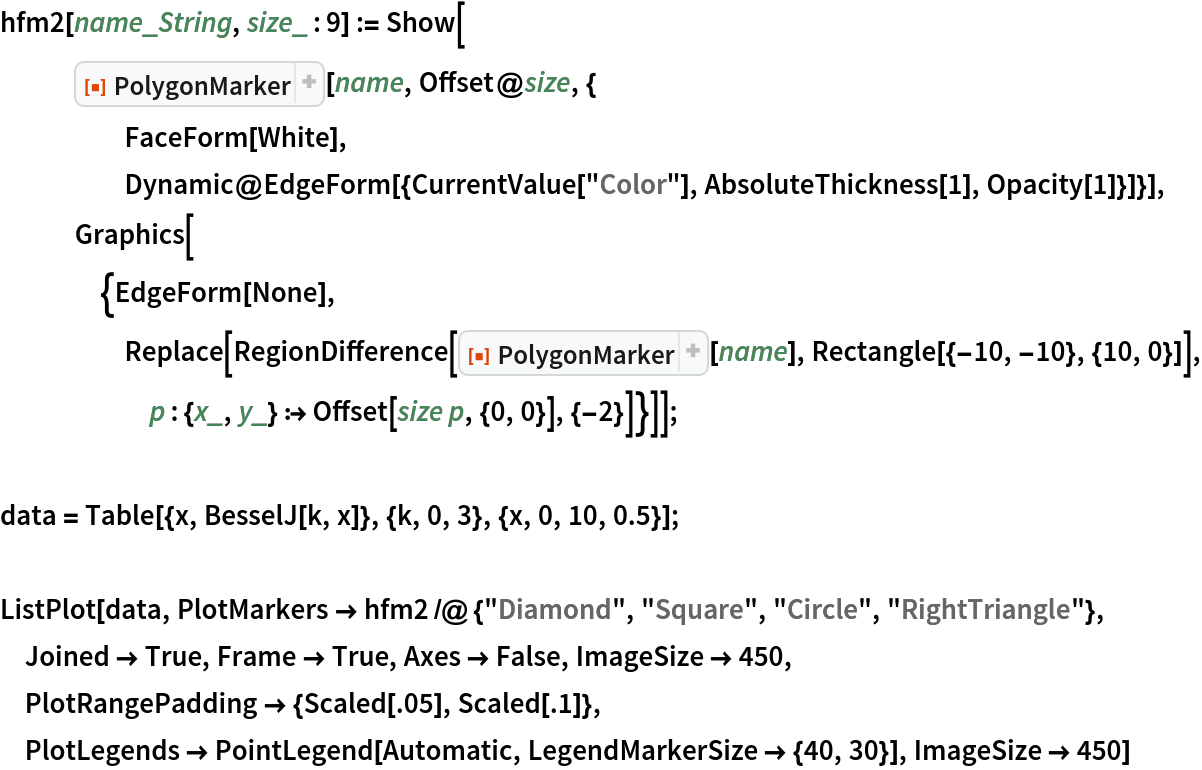Out=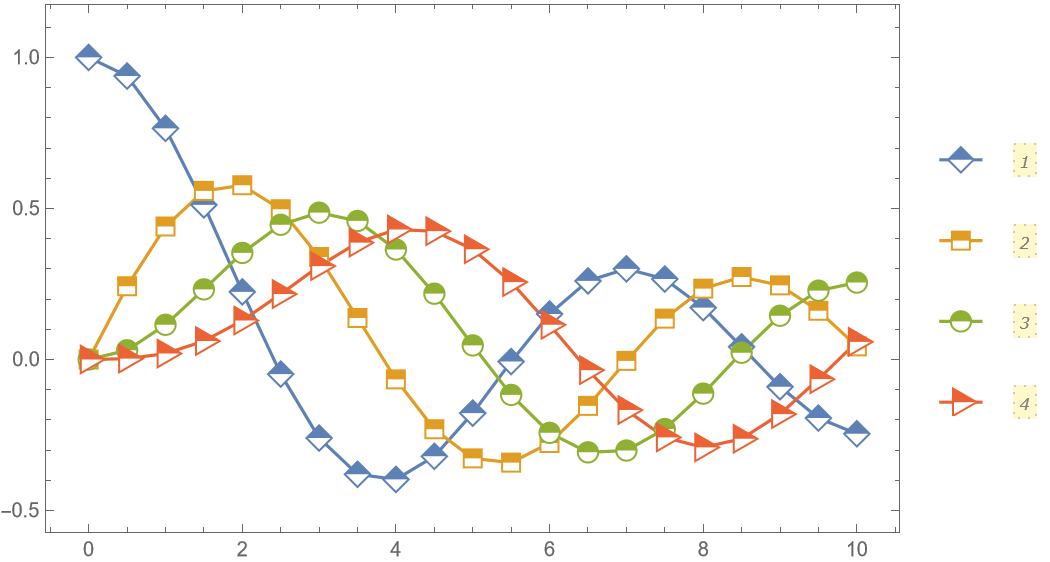In:=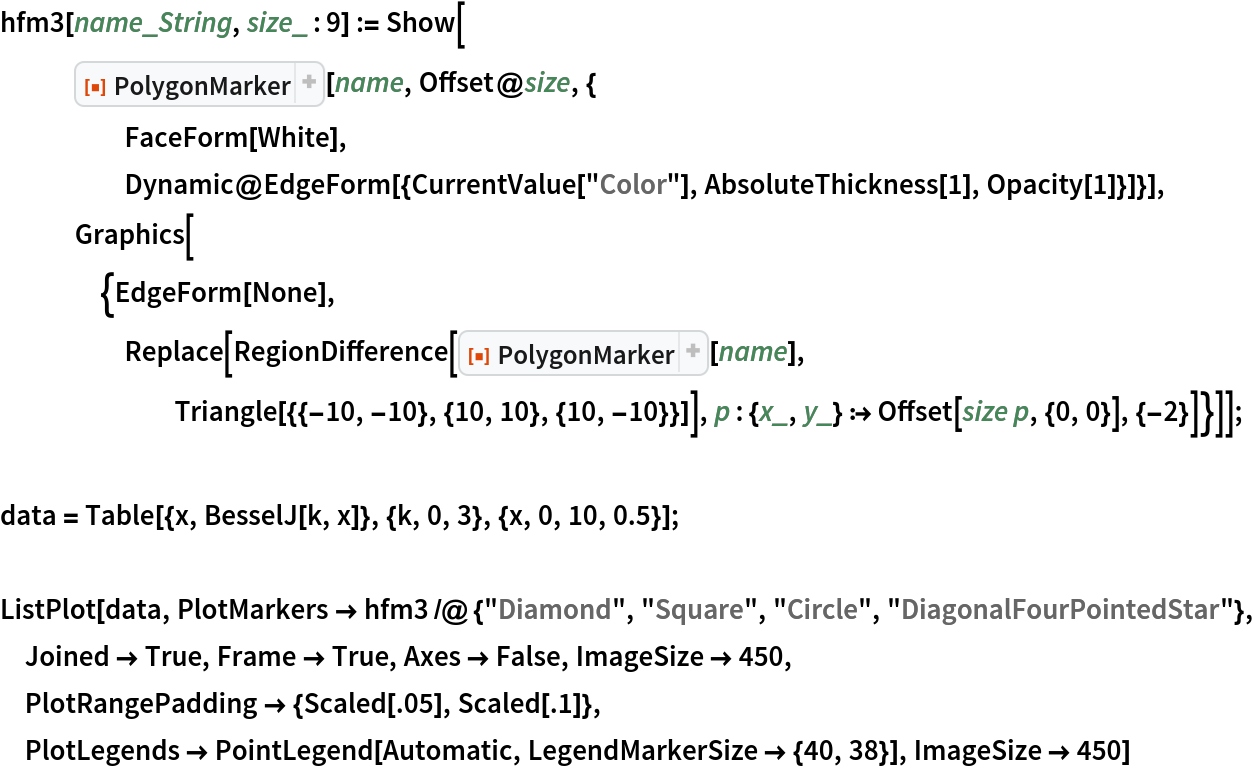Out=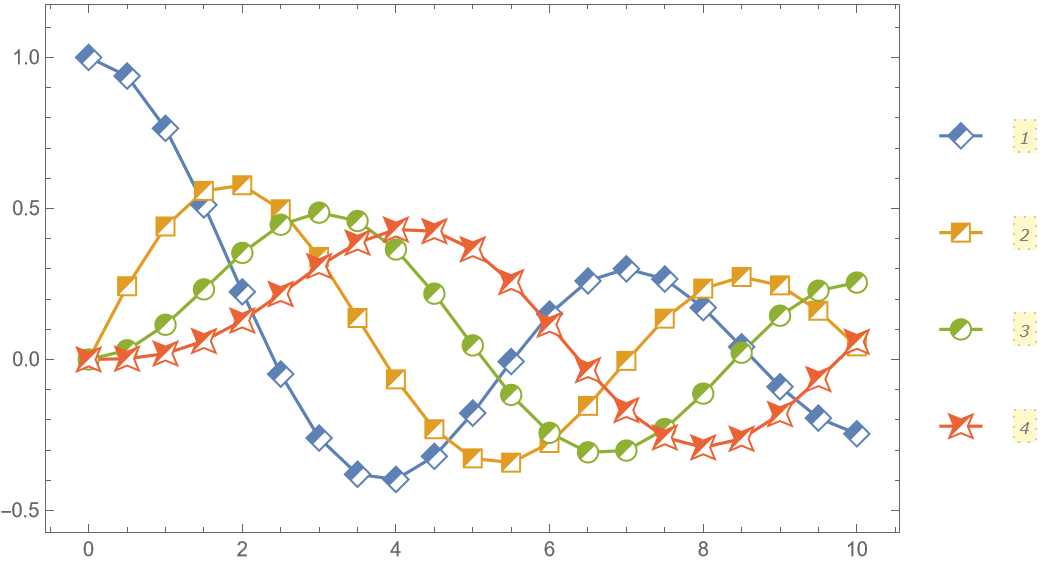Contrast markers that pick up PlotStyle and PlotTheme automatically:

 In:=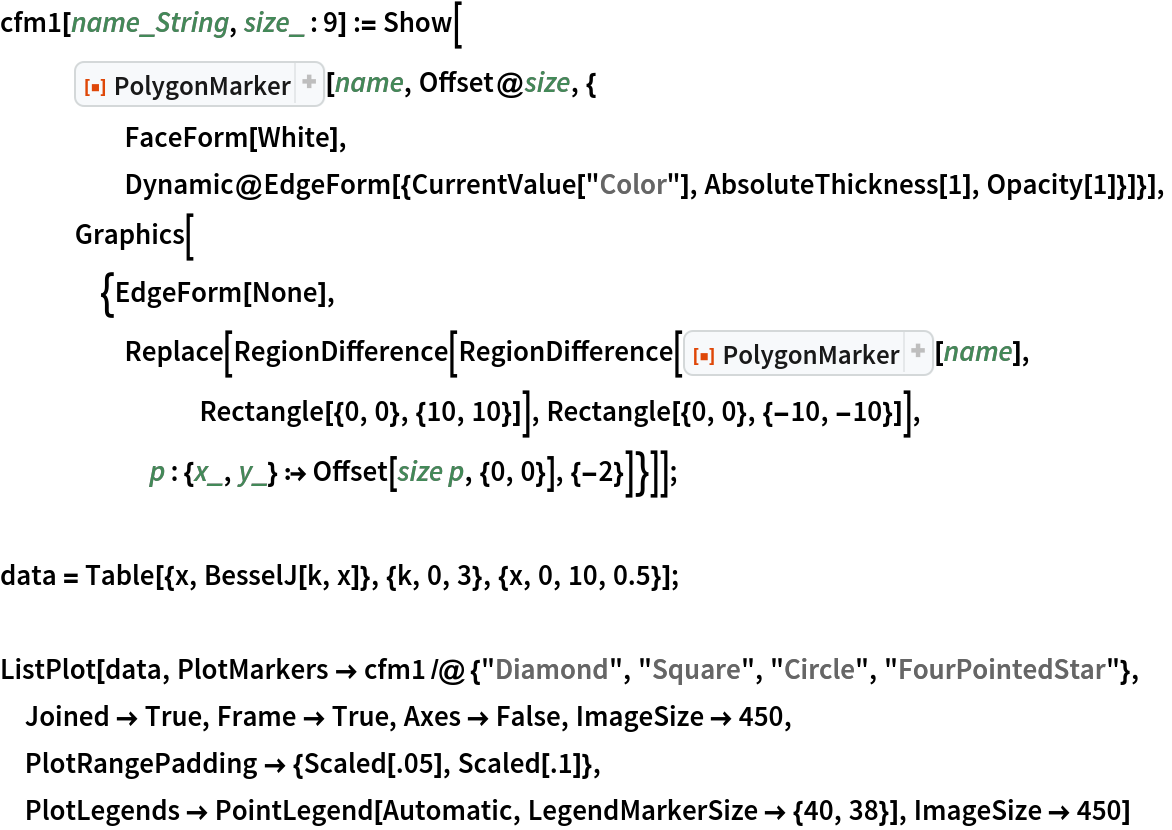Out=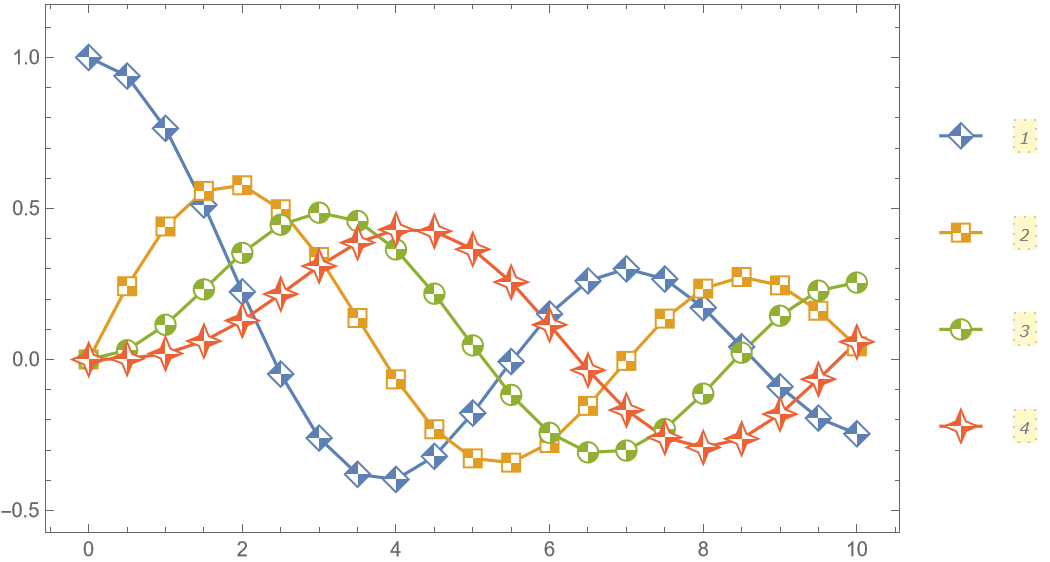In:=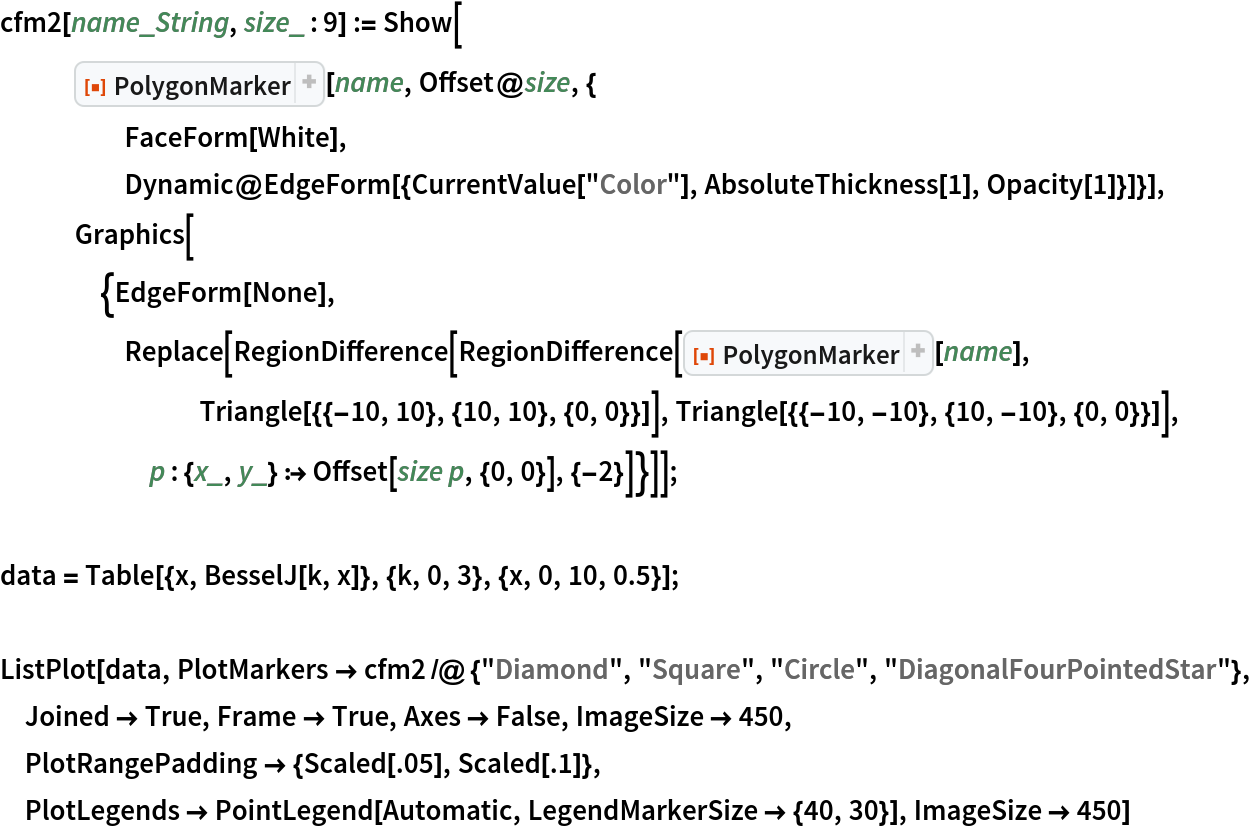Out=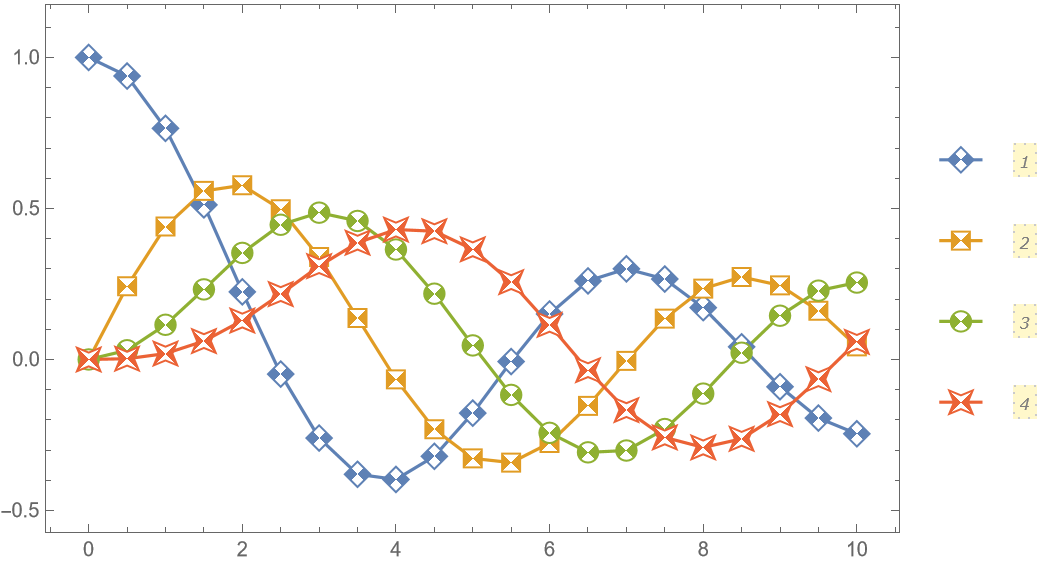Create an auxiliary function that (approximately) converts a simple glyph into a set of points suitable for PolygonMarker:

 In:=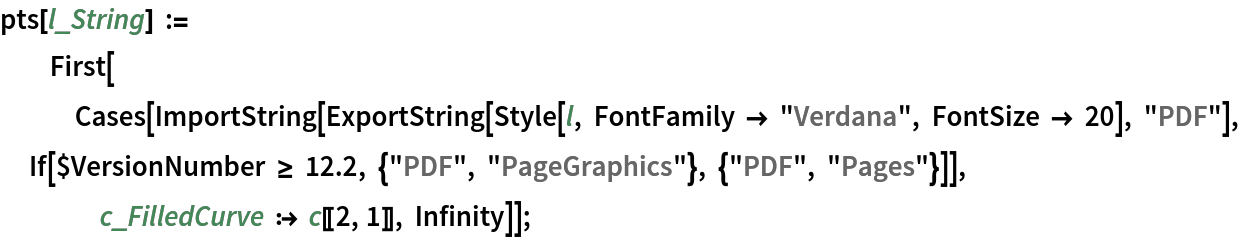Show a set of markers in use, including some created from glyphs:

 In:=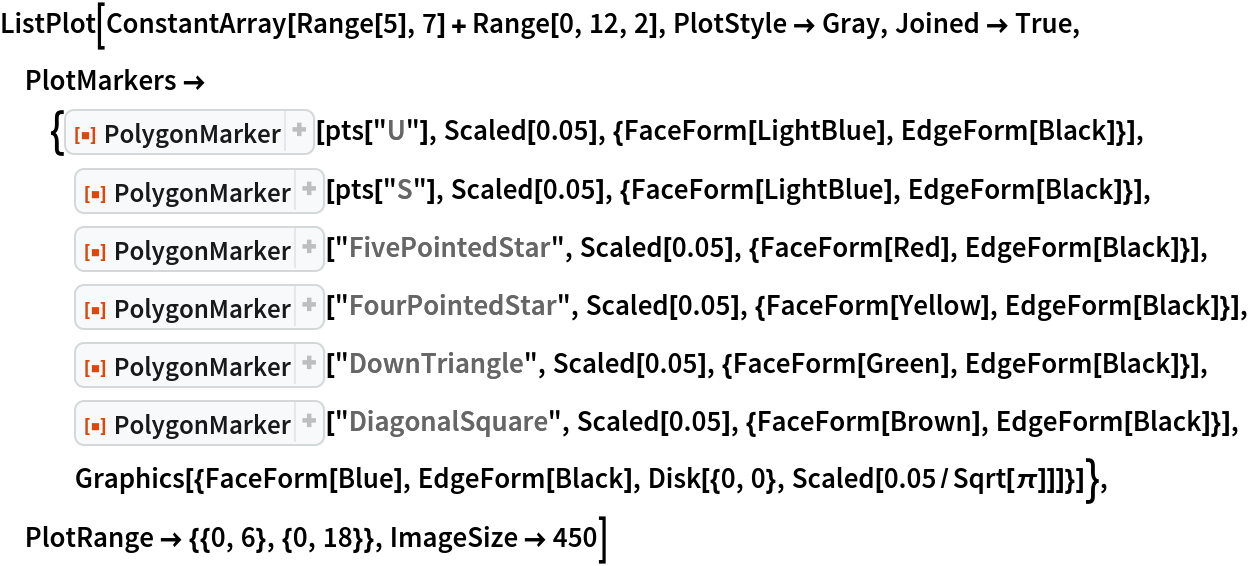Out=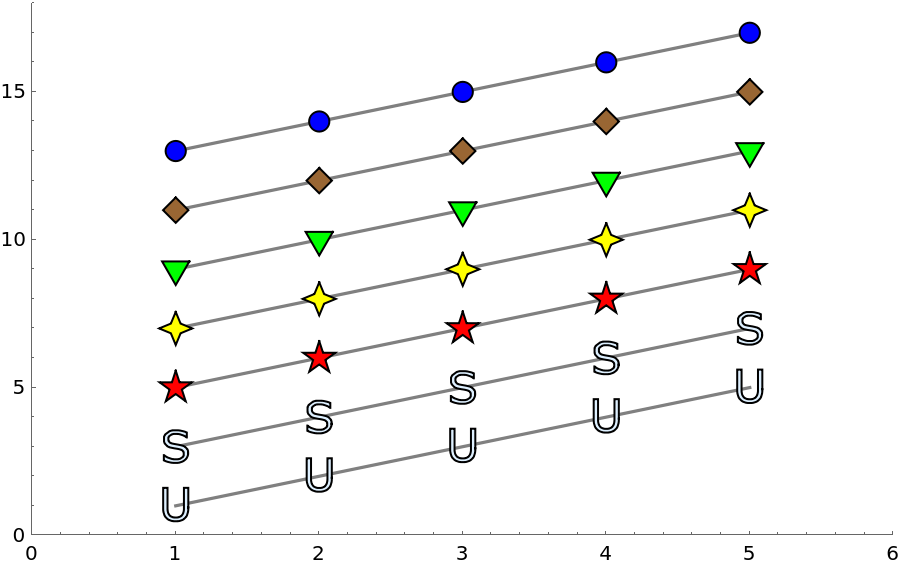Black and white plot, where the markers overlap considerably:

 In:=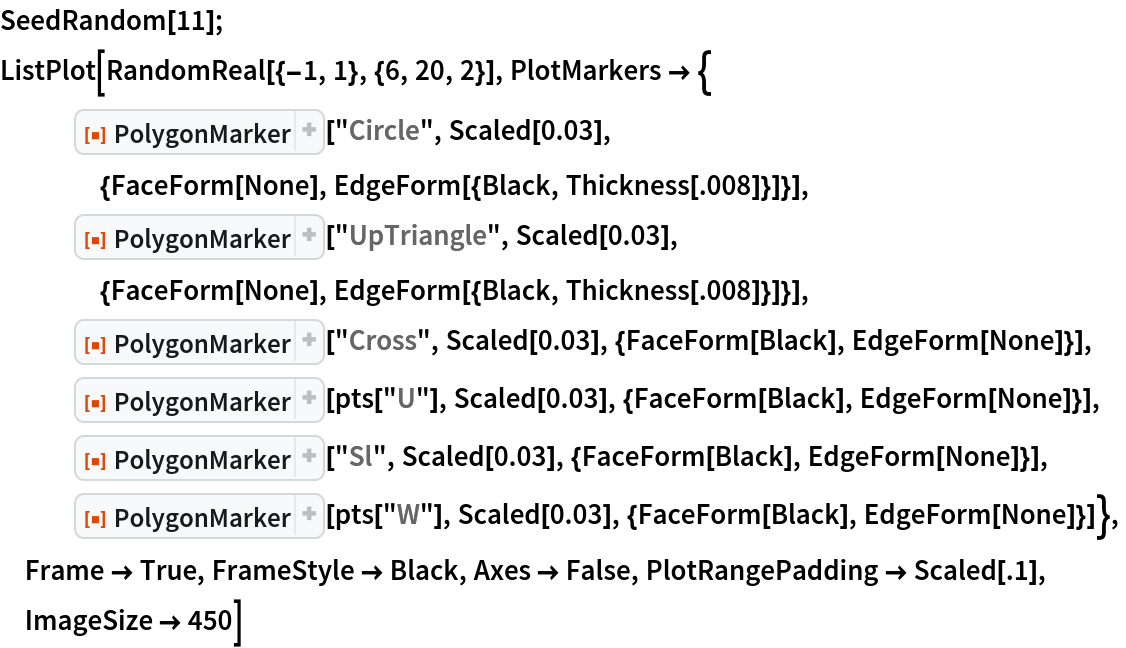Out=Use the resource functions RoundedPolygon and NotchedPolygon to make markers:

 In:=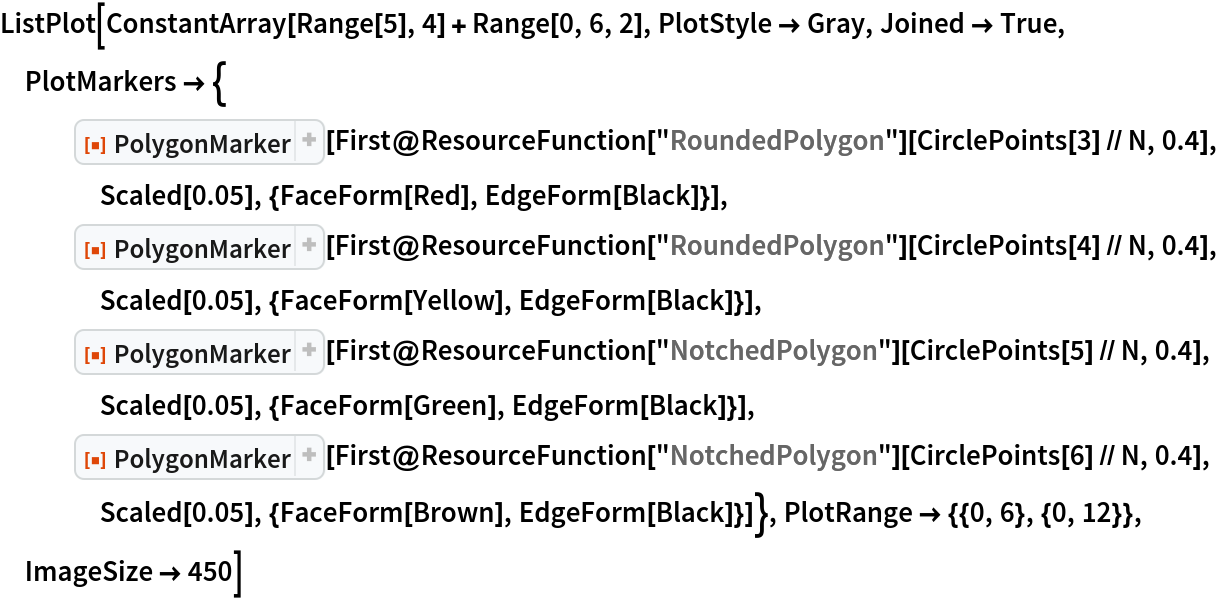Out=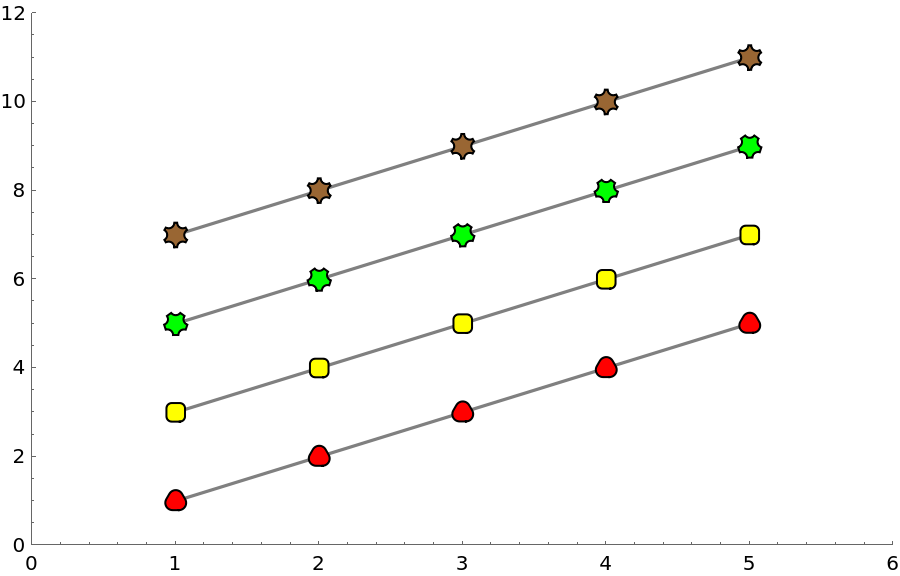AlexeyPopkov

## Version History

• 4.1.0 – 08 July 2022
• 4.0.1 – 28 February 2022
• 4.0.0 – 31 January 2022
• 3.0.1 – 16 August 2021